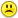+0

# A^3^2/5 x (B^10)^2 + C^20 / √D^10

0
884
11

A^3^2/5 x (B^10)^2 + C^20 / √D^10

Apr 12, 2015

#4
+5

$$\Displaystyle \text {Basic form} \\ \Displaystyle x^{3^4}\\ \Displaystyle \text {This format will work for more complex forms}\\ \Displaystyle \begin{Large} {X^{2^4^{^6^}^{^8^}^{^{Etc}}}\end{Large}\\\\ \text {Here are some other presentation forms that may be useful.}\\$$

\noindent \sqrt[\leftroot{-2}\uproot{4} 7]9876 \leftarrow \Text{Use noindent to prevent pesky indentation}\\\ \noindent \sqrt[\leftroot{-2}\uproot{4} 7]{9876}\leftarrow \Text{Note the root function covers all the numbers here. Compare the codes to see why} \\\\ \Text{Here's a method to nest "n-root" and align the "n" using "leftroot" and "uproot"}\\\\\ \sqrt[\leftroot{-2}\uproot{4} 7]{\sqrt[\leftroot{-2}\uproot{4} 5]{ (\sqrt[\leftroot{-2}\uproot{2} 9]{x^2})^3}} = Z \\\ \Text{Note the subtle difference in the exponent of 3}\\\ \sqrt[\leftroot{-2}\uproot{4} 7]{\sqrt[\leftroot{-2}\uproot{4} 5]{ (\sqrt[\leftroot{-2}\uproot{2} 9]{x^2})^{^3}}} = Z \\\ \Text{One great thing about writing great LaTex presentations: You can be dumber than a box of rocks and still look intelligent --at least for awhile. I have been getting away with it for years . . . .}

.
Apr 13, 2015

#1
+5

IF you have an exponent to an exponent, multiply the exponents together:

A^3^2  =  A^6          (B^10)^2  =  B^20         √D^10  =  (D1/2)^10  =  D^5

A^6 / 5 x B^20  =  (A6B20)/5 + C20/D5

Apr 12, 2015
#2
+5

Sorry Geno, but the first one is wrong, (A^3)^2 = A^6, but A^3^2 = A^9. Exponentiation works back to front.

Apr 12, 2015
#3
+5

Thanks Geno and Bertie,

I have always found this really confusing Bertie.  I don't think that I ever knew the rule.I am repeating what you said because I want to cement it into my head.

$$\\(A^3)^2=A^3*A^3=A^6\\\\ but\\\\$$

A^3^2  =   $$=A^{(3^2)}=A^9$$

NOW I have discovered a new (LaTex) dilema.

How do I write  A^3^2 in latex without any brackets ? This it what I get.    $$A^3^2$$

The second caret (hat)  is ignored.Apr 13, 2015
#4
+5

$$\Displaystyle \text {Basic form} \\ \Displaystyle x^{3^4}\\ \Displaystyle \text {This format will work for more complex forms}\\ \Displaystyle \begin{Large} {X^{2^4^{^6^}^{^8^}^{^{Etc}}}\end{Large}\\\\ \text {Here are some other presentation forms that may be useful.}\\$$

\noindent \sqrt[\leftroot{-2}\uproot{4} 7]9876 \leftarrow \Text{Use noindent to prevent pesky indentation}\\\ \noindent \sqrt[\leftroot{-2}\uproot{4} 7]{9876}\leftarrow \Text{Note the root function covers all the numbers here. Compare the codes to see why} \\\\ \Text{Here's a method to nest "n-root" and align the "n" using "leftroot" and "uproot"}\\\\\ \sqrt[\leftroot{-2}\uproot{4} 7]{\sqrt[\leftroot{-2}\uproot{4} 5]{ (\sqrt[\leftroot{-2}\uproot{2} 9]{x^2})^3}} = Z \\\ \Text{Note the subtle difference in the exponent of 3}\\\ \sqrt[\leftroot{-2}\uproot{4} 7]{\sqrt[\leftroot{-2}\uproot{4} 5]{ (\sqrt[\leftroot{-2}\uproot{2} 9]{x^2})^{^3}}} = Z \\\ \Text{One great thing about writing great LaTex presentations: You can be dumber than a box of rocks and still look intelligent --at least for awhile. I have been getting away with it for years . . . .}

Nauseated Apr 13, 2015
#5
0

Thanks Nauseated,

Could you please include the code for each bit.

Maybe separate it up so we can see the code then the output, then the next bit of code and the output.

It would be great it you did that for us.  :))

Apr 13, 2015
#6
0

I can, but it will clutter up everything. Just mouse over the Latex and the code window pops up. Select and copy that code and paste it in an editor to play with it.

It’s as easy as

$${\pi}$$

.
Apr 13, 2015
#7
0

No Nauseated,I cannot copy the content of the boxes successfully especially when the content is long.

I cannot see what you have done.Apr 13, 2015
#8
0

I have tried to reproduce it in the LaTex thread but some of it won't work properlyhttp://web2.0calc.com/questions/latex#r61

Thanks for sending me the codeApr 13, 2015
#9
0

I’ll make a separate post for it. In the mean time, here’s how to copy the code. It’s not difficult.

There are other ways to do this, but this way is fast and easy to explain.

1) Bring up the “code box” by mousing over the Latex,.

2) Move the mouse pointer over the box and right click. A dialogue box will appear.

3) The dialogue box will have the command “select all.” One of the letters will be underlined –for most browsers it is the “a”. Press the “a” on the key board. The text code in the box will highlight blue.

4) Right click again, and press “c” for copy. All the code in the box is now copied to the buffer. This text can now be pasted anywhere (where text can be pasted): a word editor, a Latex editor, or another post.

There is one thing to watch for: the mouse pointer needs to stay on the text box while performing these commands. If the mouse moves off the text box it won’t work. This is why you have to press the letter key –instead of moving the mouse.

Hummm… It looks like you figured out most of it . . . .

I’ll post follow-ups on the Latex thread.

Apr 13, 2015
#10
0

Thanks Nauseated

Apr 13, 2015
#11
0

This does not work for me Nauseated!   I do not get "select all"

2) Move the mouse pointer over the box and right click. A dialogue box will appear.

3) The dialogue box will have the command “select all.” One of the letters will be underlined –for most browsers it is the “a”. Press the “a” on the key board. The text code in the box will highlight blue.

Apr 13, 2015